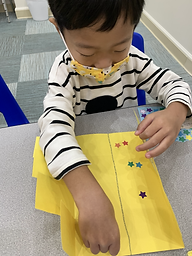Ms. Kayla

Target 1​

Lesson Type:

Continuation

Number Operation

:

Number System

Understand that numbers have an order (limited to numbers 0 to 50).

1:

Understand that the number sequence does not change, even when some numbers are missing from a given sequence.

2:

Understand that the last number counted depicts the quantity of the objects.

Pre-K

Vocabulary:

Count, Zero, One, Two, Three, Four, Five, Six, Seven, Number

Activities:

1. Students filled a flipchart that represented numbers 0-7.

2. Students identified a number and represented it with stickers.Home Exploration

Guiding Questions:Absent Students:

Target 2

:

1:

Identify simple geometric shapes in a variety of colors, sizes, and orientations.

2:

Independently identify squares, rectangles, circles, and triangles.

3:

Identify basic geometric shapes that best represent real-world objects.

Pre-K

Vocabulary:

Shape, Circle, Triangle, Rectangle, Square

Activities:

1. Students discussed the geometric shapes seen in every day objects (sun, house, tv, couch, tree, etc.).

2. Students represented the different shapes with a simple drawing.Home Exploration

Guiding Questions:Target 3

:

Vocabulary:

Activities:Home Exploration